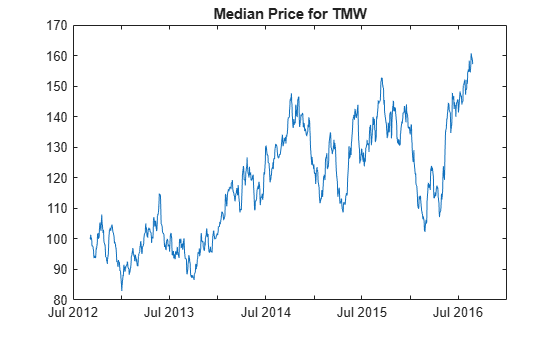# medprice

Median price from the series of high and low prices

## Syntax

``MedianPrice = medprice(Data)``

## Description

example

````MedianPrice = medprice(Data)` calculates the median prices from the series of high and low prices. The median price is the average of the high and low prices for each period.```

## Examples

collapse all

Load the file `SimulatedStock.mat`, which provides a timetable (`TMW`) for financial data for TMW stock.

```load SimulatedStock.mat MedianPrice = medprice(TMW); plot(MedianPrice.Time,MedianPrice.MedianPrice) title('Median Price for TMW')```## Input Arguments

collapse all

Data for high and low prices, specified as a matrix, table, or timetable. For matrix input, `Data` is an `M`-by-`2` matrix of high and low prices stored in the corresponding columns. The columns can be in either order because the median price is the average of the high and low prices for each period. Timetables and tables with `M` rows must contain a variable named `'High'` and `'Low'` (case insensitive).

Data Types: `double` | `table` | `timetable`

## Output Arguments

collapse all

Median price series, returned with the same number of rows (`M`) and the same type (matrix, table, or timetable) as the input `Data`.

 Achelis, S. B. Technical Analysis from A to Z. Second Edition. McGraw-Hill, 1995, pp. 177–178.